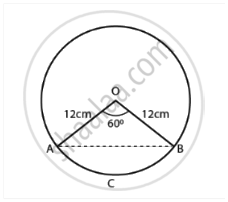# Find the area of the segment of a circle of radius 12 cm whose corresponding sector has a central angle of 60° (Use π = 3.14). - Mathematics

Sum

Find the area of the segment of a circle of radius 12 cm whose corresponding sector has a central angle of 60° (Use π = 3.14).

#### Solution

Radius of the circle = r = 12 cm

OA = OB = 12 cm

∠AOB = 60°   .......(GIven)As triangle OAB is an isosceles triangle,

∠OAB = ∠OBA = θ  .....(Let)

And sum of interior angles of a triangle is 180°,

θ + θ + 60° = 180°

2θ = 120°

θ = 60°

Therefore, the triangle AOB is an equilateral triangle.

AB = OA = OB = 12 cm

Area of the triangle AOB = (sqrt(3)/4) xx a^2.

Concept: Areas of Sector and Segment of a Circle
Is there an error in this question or solution?

#### APPEARS IN

NCERT Mathematics Exemplar Class 10
Chapter 11 Area Related To Circles
Exercise 11.4 | Q 4 | Page 133
Share